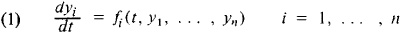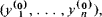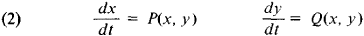# Periodic Solutions

The following article is from The Great Soviet Encyclopedia (1979). It might be outdated or ideologically biased.

## Periodic Solutions

Periodic solutions of equations are solutions that describe regularly repeating processes. In such branches of science as the theory of oscillations and celestial mechanics, periodic solutions of systems of differential equationsare of special interest. A periodic solution yi = Φi (t) of (1) consists of periodic functions of t that have the same period. In other words,

Φi (t + τ) = Φi (t)

for all t and for some τ > 0; τ is called the period of the solution. If the system (1) is autonomous—that is, the functions fi = Fi (y1, …, yn), i = 1, …, n, do not explicitly depend on t —then to the periodic solutions there correspond closed trajectories in the phase space (y1, …, yn). A degenerate form of such trajectories are the equilibrium, or critical, pointswhereThe critical points correspond to trivial (constant) periodic solutions. The problem of finding nontrivial periodic solutions has been solved only for special types of differential equations.

Systems of two equationsare of particular importance in the theory of nonlinear oscillations. Their phase space is the xy-plane. The critical points of system (2) can be found from the system of equations P (x, y) = 0, Q (x, y) = 0. System (2) does not admit of nontrivial periodic solutions if P′x (x, y) + Q′y (x, y) = 0 (Bendixson’s criterion).Figure 1

The usual method of finding nontrivial periodic solutions of system (2), if they exist, is to construct a bounded annular region K (see Figure 1) such that all trajectories enter it as t → + ∞ or t → – ∞. If k does not contain critical points of system (2), it necessarily contains a closed trajectory that corresponds to a nontrivial periodic solution (the Poincaré-Bendixson theorem). Another approach to finding periodic solutions is provided by the study of the behavior of solutions in neighborhoods of critical points: in the neighborhood of a center, the integral curves of system (2) are closed and correspond to nontrivial periodic solutions.

### REFERENCES

Nemytskii, V. V., and V. V. Stepanov. Kachestvennaia teoriia differentsial’nykh uravnenii, 2nd ed. Moscow-Leningrad, 1949.
Andronov, A. A., A. A. Vitt, and S. E. Khaikin. Teoriia kolebanii, 2nd ed. Moscow, 1959.
Stoker, J. Nelineinye kolebaniia v mekhanicheskikh i elektricheskikh sistemakh, 2nd ed. Moscow, 1953. (Translated from English.)
References in periodicals archive ?
where H = [pi]/2(1-k), [PHI]([THETA]) - a periodic function of the variable [THETA] with period 2[pi]/[sigma] has periodic solutions with the same period if
Omari, "Nonresonance with respect to the Fucik spectrum for periodic solutions of second order ordinary differential equations," Nonlinear Analysis: Theory, Methods & Applications, vol.
 discussed the existence and stability of the periodic solutions of (1) and obtained the sufficient conditions which guaranteed the existence and stability of the periodic solutions for (1) from a particular one.
Considering the periodic variation and pollution exposure of epidemic models and exploring the existence of stochastic periodic solutions are meaningful to predict and control the outbreaks of infectious diseases.
(6) In the paper titled "Odd Periodic Solutions of Fully Second-Order Ordinary Differential Equations with Superlinear Nonlinearities," the authors were concerned with the existence of periodic solutions for the fully second-order ordinary differential equation, where the nonlinearity is continuous and (t, x, y) is 2[pi]-periodic in t.
On the one hand, it follows from the classical results about random periodic solutions of SDEs [1, 4] that the numerical simulation of random periodic solution has been performed by the author; see  and references therein.
When W(t, q) is asymptotically linear with respect to q both at origin and at infinity, the existence of nontrivial periodic solutions of system (2) has been proved in .
For parameters on the line AB in Figure 5, Equation (6) has periodic solutions with period of 4[pi] regardless of whether [delta] < 0.
The principle of the Lindstedt method is to find approximations for periodic solutions, by convergent series using the expansion theorem and the periodicity of the solution [13, 14].
In the study , the creation of periodic orbits associated with grazing bifurcations in the models of impacting systems and some sufficient conditions are obtained for the existence of a family of periodic solutions. In , two distinct types of grazing bifurcations are taken into account.
One paper studies the stability analysis of the periodic solutions of some kind of predator-prey dynamical systems.
We then analyze the global stability of the two boundary equilibria, the local asymptotic stability and Hopf bifurcation of the positive equilibrium and also the stability of the bifurcating periodic solutions. Moreover, we perform numerical simulations to illustrate some of the theoretical results we obtain and also illustrate how the factors such as the immune response to infected cells and viruses and the proliferation of hepatocytes affect the dynamics of the model under time delay.

Site: Follow: Share:
Open / Close